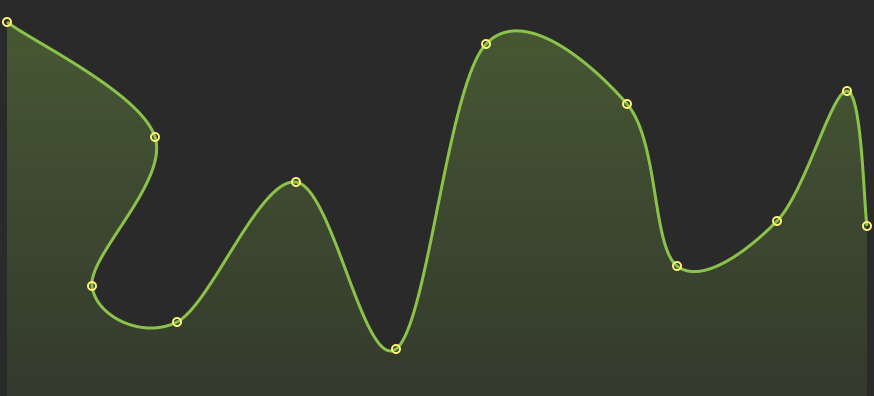## cubic_spline

Function that calculates curve points for cubic spline

### 19 releases(1 stable)

 1.0.0 Dec 14, 2020 Nov 4, 2020 Jul 25, 2020 Feb 8, 2020 Jun 20, 2019

#275 in Data structures

Used in aoer-plotty-rs

45KB
737 lines

# cubic_spline

Interpolation method for computation of cubic spline points within the range of a discrete set of known points.## Example

``````use cubic_spline::{Points, Point, SplineOpts, TryFrom};

fn main() {
let source = vec![(10.0, 200.0), (256.0, 390.0), (512.0, 10.0), (778.0, 200.0)];

let opts = SplineOpts::new()
.tension(0.5);

let mut points = Points::try_from(&source).expect("expect valid points but");
let result = points.calc_spline(&opts).expect("cant construct spline points");

assert_eq!(result.get_ref().len(), 49);

let inner_vec: &mut Vec<Point> = points.get_mut();
inner_vec.push(Point::new(7.7, 1.3));
inner_vec.x += 0.79;
inner_vec.last_mut().iter_mut().for_each(|mut p| {p.tension = Some(0.7);});

points.invert_vertically(400.0);

assert_eq!(points.get_ref().y, 10.0);

let calculated_points = points
.calc_spline(&opts.num_of_segments(33))
.unwrap();

assert_eq!(calculated_points.into_inner().len(), 133);

}
``````

For information on how a curve can be constructed and which points to accept, see the appropriate structures.

## Custom points

If you already have some points you can implement `From` trait for `Point` struct and pass your points directly.

### Example

``````use cubic_spline::{SplineOpts, Point, Points};

#[derive(Default)]
struct MyPoint {
vertical: u8,
horizontal: u8,
color: String,
}

impl<'a> From<&'a MyPoint> for Point {
fn from(p: &'a MyPoint) -> Self {
Point::new(&p.horizontal as f64, &p.vertical as f64)
}
}

fn main() {
let my_points: Vec<MyPoint> = vec![MyPoint::default(),MyPoint::default()];
let spline = Points::from(&my_points)
.calc_spline(&SplineOpts::default())
.unwrap();

assert_eq!(spline.get_ref().len(), 17);
}

``````

## Use in Javascript

It also compiled as wasm module. And you can use it in your js code but not completely. Now available only one function

``````import { getCurvePoints } from 'cubic-spline-rs'

const NUM_OF_SEGMENTS = 22

const points = [10.0, 200.0, 256.0, 390.0, 512.0, 10.0, 778.0, 200.0]

const curvePoints = getCurvePoints( points, {
num_of_segments: NUM_OF_SEGMENTS, // *optional
// tension: 0.5, // *optional
// ...
} )

``````

If you want to draw result points to canvas - code like this:

``````const ctx = getMyCanvas2DContext()

ctx.beginPath()
ctx.lineWidth = 3
ctx.strokeStyle = '#ffcc00'

ctx.moveTo(curvePoints, curvePoints)
const length = curvePoints.length - 1
for (let i = 2; i < length; i += 2) {
ctx.lineTo(curvePoints[i], curvePoints[i + 1])
}

ctx.stroke()
ctx.closePath()
``````

### Options

Name Type Default Description
tension `f64` `0.5` Tension
num_of_segments `u32` `16` Number of calculated points between known points
hidden_point_at_start `Option<(f64,f64)>` `None` A point that will not be drawn, but the beginning of the graph will bend as if it is there.
hidden_point_at_end `Option<(f64,f64)>` `None` A point that will not be drawn, but the end of the graph will bend as if it is there.
``````use cubic_spline::{SplineOpts};

fn main() {
let options = SplineOpts::new()
.tension(0.6)
.num_of_segments(54)
// .hidden_point_at_start((1.2, 3.1))
// .hidden_point_at_end((397.9, 105.5))
;

}

``````